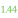# LOG2E

 Block Group: Constants Icon:The LOG2E block is a Number block. When the LOG2E block is created, the LOG2E block holds the mathematical constant for the base-2 logarithm of the constant e.

For information on using dataflow blocks, see Dataflow.

## Input/Output Property

The following property of the LOG2E block can take input and give output.

• value (number)

value sets and returns the number held by this LOG2E block. When a LOG2E block is created, the value property holds the mathematical constant for the base-2 logarithm of the constant e.

## Example

The following image demonstrates an example of the LOG2E block.